## Heat Temperature and Thermal Expansion Exam2 and Problem Solutions

Heat Temperature and Thermal Expansion Exam2 and  Problem Solutions

1. If Celsius thermometer shows the temperature of air 300C, find the temperature of air in Fahrenheit thermometer.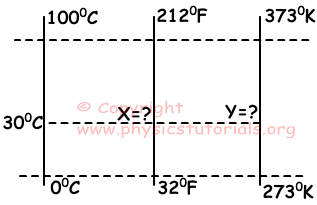T(K)=T(C)+273

T=30+273=3030K

C/100=(F-32)/180

30/100=(F-32)/180

F=860F

2. Find heat required to make 5g ice at -200C to water at 300C. (cice=0,5cal/g.0C, Lice=80cal/g, cwater=1cal/g.0C)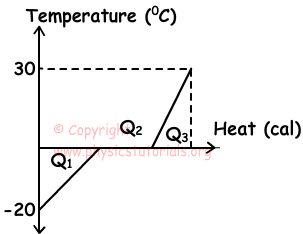Heat required to make ice at -200C to ice at 00C ;

Q1=m.cice.ΔT=5.0,5.20

Q1=50cal.

Heat required to make it melt;

Q2=m.Lice=5.80

Q2=400cal.

Heat to make it water at 300C;

Q3=m.cwater.ΔT=5.1.30

Q3=150cal

Qtotal=Q1+Q2+Q3=50+400+150=600cal

3.  Two taps fill the water tank with different flow rates. Tap A fills the tank in 1 hour and tap B fills the tank in 3 hour If we open two taps together, find the final temperature of the water in the tank.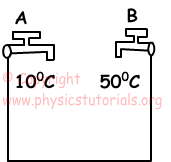Flow rates of taps;
VA=3VB

3m.c.(T-10)=m.c(50-T)

T=200C

4. When we decrease the temperatures of the rods ΔT, relation between the final lengths of rods becomes; L1<L2<L3. Find the relation between αA, αB and αC.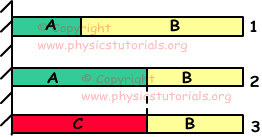Since change in length of the rod 1 is larger than the rod 2;

αAB

Since change in length of the rod 2 is larger than the rod 3;

αCA

αCAB

5. Length vs. temperature graph of A, B and C is given below. Find the relation between λA, λB and λC.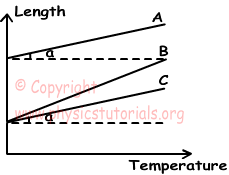Slope of the graph=ΔL/ΔT=L0

Initial length of A is larger than C but slopes of them are equal, so;

λCA

B and C  have same length but slopes of them are different.

λBC

λBCA

Author: